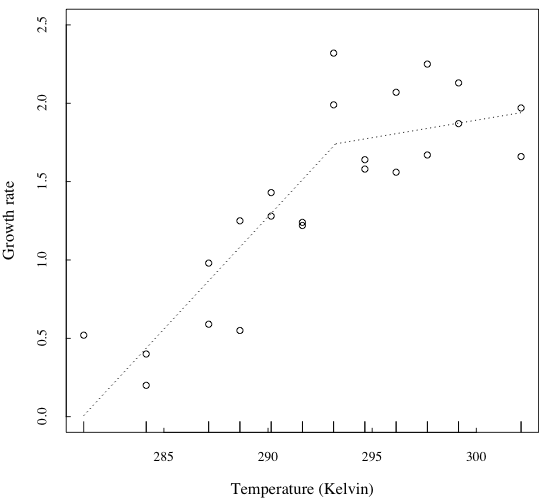# Testing the Metabolic Theory of Ecology: a worked example using R

[Download the simulated data and the R script used in the following example]

Many biological variables depend on the size of the organisms and on the environmental temperature. For example, large organisms tend to grow more slowly, and live longer, than small ones. On the other hand, organisms tend to grow faster in warm climates compared to cold ones. Moreover, small organisms and organisms from warm climates consume more resources (per mass unit) than large organisms and those living in cold climates.

The effect of body mass and temperature at the individual level reflects at the ecological level, in variables such as the intrinsic growth rate of populations, the strength of competition and predator-prey interactions (e.g. Vucic-Pestic et al. 2011), and so on.

Scientists suggested that these patterns are explained by the role that body mass and temperature play on the metabolism of individuals, namely the ensemble of the chemical reactions that keep them alive. In 2004, Brown et al. summarized and formalized what they called the Metabolic Theory of Ecology (MTE), centered on the following equation:

(Eq.1)            I=i0M3/4e-E/kT

Where:

• I is the metabolic rate, or any metabolism-driven biological variable
• i0 is the value of I for temperature = 0 K, or for any temperature chosen as reference
• M is the body mass
• T is temperature in Kelvin
• k is the Boltzman constant
• E is the activation energy.

It is noteworthy that, if we are studying the temperature response of a single species, we can assume i0 * M3/4 to be a constant, A, across temperature; hence, the Eq.1 can be simplified as:

(Eq.2)            I=Ae-E/kT

Which is the Arrhenius equation. The Arrhenius equation is based on the fact that most chemical reactions, including those involved in metabolism, do not happen spontaneously because of an energetic threshold called Activation Energy (E). As the temperature increases there is more energy available to overcome the energetic threshold represented by E, hence reactions develop at an exponentially faster rate.

Brown et al. (2014) suggest that the temperature-driven changes on individuals’ metabolism lead to ecological variables to vary with temperature in a similar, exponential fashion. The exponential relationship should hold up to an optimum temperature Topt, beyond which individual fitness drops precipitously (see the figure below).Thermal response curve obtained using the model “Lactin-2” proposed by Lactin et al. in 1995 (see R script for details.). For a possible theoretical explanation of why we observe such left-skewed relationships between biological variables and temperature, see Amarasekare & Savage 2012.

How to test the Metabolic Theory of Ecology (MTE)? One way is to experimentally grow an organism at a range of temperatures, record its intrinsic growth rates, and see if they show an exponential correlation to temperature. We can do this using the freely available software R.

Let’s imagine that we did this experiment with a microorganism, say the imaginary bacterium Bacillus phantasticus. This is what we observe:Estimated values of growth rate for each of the 12 experimental temperatures (between 8°C and 29°C). The dotted line represent the output of a segmented regression, showing a break point around 293 K (20°C). See R script for details.

We notice that the intrinsic growth rate r is positively correlated to temperature up to a point, beyond which it reaches a plateau. In order to test the MTE, we need to fit Eq. 2 on the pre-plateau data points. We could select the threshold by eye, or we can do it using segmented regression (for example, function segmented in the R package segmented). We see that the threshold is 293.3 K, i.e. 19.85°C. We can then fit Eq. 2 on the pre-threshold subset of our data points. I do this using the R function nls():

``````start.pars <- c(pre.exp=1, aer=0.67)
# starting points for nls() to start searching the parameter values from
nls1 <- nls(y ~ pre.exp * exp(aer*(Kelvin - T.ref)/(boltz*Kelvin*T.ref)),
start=list(pre.exp= start.pars, aer= start.pars),
data=dat[dat\$Kelvin < 293.3,]
)
summary(nls1)``````

This is the outcome:

``````Formula: y ~ pre.exp * exp(aer * (Kelvin - T.ref)/(boltz * Kelvin * T.ref))

Parameters:
Estimate Std. Error t value Pr(>|t|)
pre.exp  0.84711    0.09701   8.732 2.81e-06 ***
aer      1.25442    0.21525   5.828 0.000115 ***
---
Signif. codes:  0 ‘***’ 0.001 ‘**’ 0.01 ‘*’ 0.05 ‘.’ 0.1 ‘ ’ 1

Residual standard error: 0.274 on 11 degrees of freedom

Number of iterations to convergence: 6
Achieved convergence tolerance: 9.568e-07
(1 observation deleted due to missingness)``````

The parameter “aer” gives a measure of the steepness of the exponential curve. Its value is 1.25 and, if the MTE holds, it should be an estimate of the activation energy (in electronVolts, eV) of the main metabolic reaction of B. phantasticus (likely to be the oxydation of carbohydrates, i.e. respiration).Simplified MTE model (i.e. Arrhenius model) fitted on the experimental data. See R script for details.

Is the MTE model describing the observed data better than a linear regression? We can compare the two model using tha Akaike Information Criterion, AIC:

``AIC(nls1, linear_model)``

The MTE model gets the lowest AIC, indicating that it is the best model of the two.

What else could we do? To begin, Lactin et al., Amarasekare and Savage all described the situation of a strongly left-skewed thermal dependence with a narrow Topt. Our experiment shows that Topt and Tmax of B. phantasticus are quite far from each other. In fact, Tmax is beyond the range of temperatures used in the experiment. To really assess the skewedness of the thermal response of the species’ r we should run a new experiment on a broader temperature range.

.

.

BIBLIOGRAPHY